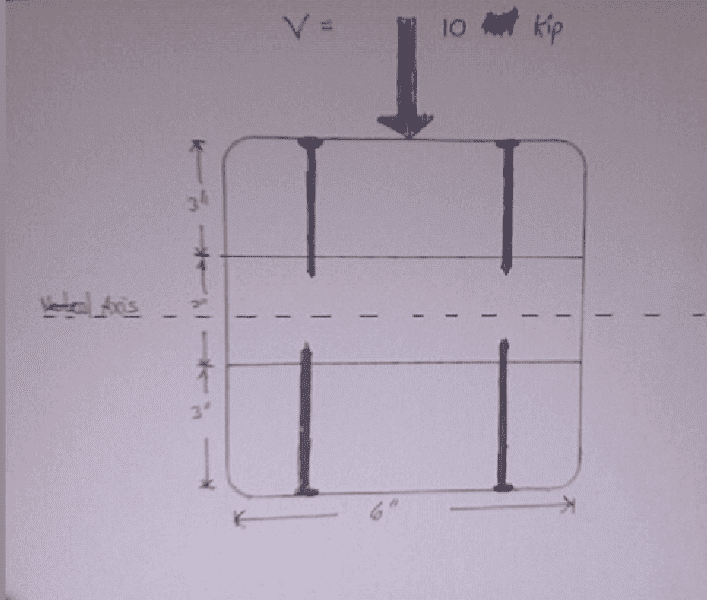We are doing shear flow problems and my instructor has been unable to explain the topic in a general enough way to apply effectively to all problems. Our (my class) difficulty is understanding in the formula q=QV/I where the variable Q=yA' what to use for A'.

It seems to vary so drastically from problem to problem that there is no set way to find it.

Will someone please explain to me what to use for A' in this problem:

The three boards are nailed together as shown. How should the nails be spaced if each nail can resist 100 lbs?I was thinking of using $$q=\frac{F}{spacing}=\frac{QV}{I}$$ and only using the area of the top board. This would get me the spacing if there were only two nails. Then I could multiply that number by two to get it for four nails since it is symmetrical about the neutral axis.

Does this sound right?

I hate to be a pain. But since no one ever checks this forum, I am going to bump this thread every few minutes until it gets some views. I figure if I keep it in the "header" someone has to look at it eventually.

Sorry to be a PITA, but I really need to get this!

Homework Helper
Gold Member
Slow down, chief! A sure fire way to not get help is to keep posting before an answer, because helpers often look for problems with 0 responses, not multiple ones.
Anyway, the value for A', for calcualting shear in the upper nails,is the area of the top board. Similarly, for calculating shear in the bottom nails, you have to use A' for the bottom board. You can't just spread the load amongst both sets, you've got to look at each pair separately (they each see the same shear flow because of symmetery). Otherwise, your approach seems OK. Check your value for V or the allowable nail force...one or the other seems too high or low.

Staff Emeritus
Is this a composite beam? Besides the normal load, is there a lateral load as well?

Is one interested in the shear load along the nails (between nails and wood) or through the cross-section of the nails?

It is actually shear flow which is defined as force per unit length along a longitudinal plane. http://en.wikipedia.org/wiki/Shear_flow

What is tricky is finding out which Q to use. It is different on a case by case basis. I think that my assumption, though, was correct. To analyze the shear flow in each nail, I could use the top board (it is three boards nailed together by the way) and find the shear flow in two nails and then divide that number by two (since the beam is symetrical).

Homework Helper
Gold Member
It is actually shear flow which is defined as force per unit length along a longitudinal plane. http://en.wikipedia.org/wiki/Shear_flow

What is tricky is finding out which Q to use. It is different on a case by case basis. I think that my assumption, though, was correct. To analyze the shear flow in each nail, I could use the top board (it is three boards nailed together by the way) and find the shear flow in two nails and then divide that number by two (since the beam is symetrical).
I think you may have it right, but unless you show some numbers, I can't be sure. When you calculate the shear flow for at the joint between the top and middle board, you must divide that flow by 2 to get the shear in each nail at the top. To get the shear in each nail at the bottom, you calculate the shear flow at the joint between the bottom and middle board, and divide that flow by 2. Is this what you are saying? But when i run your numbers, i get a very impractical small nail spacing along the length of the member in order to keep the nail shear force to 100 pounds per nail; that's why I asked you to check your input values.

To get the shear in each nail at the bottom, you calculate the shear flow at the joint between the bottom and middle board, and divide that flow by 2. Is this what you are saying?

Yes. That is. Sweet!

But when i run your numbers, i get a very impractical small nail spacing along the length of the member in order to keep the nail shear force to 100 pounds per nail; that's why I asked you to check your input values.

Yeah, I made up the problem and pulled the numbers out of the sky. I left my text at home and the question was urking me... so I just made one up.

Thanks. I will probably revive this thread when I find another problem with less forgiving geometry. Those are the ones that kill me.